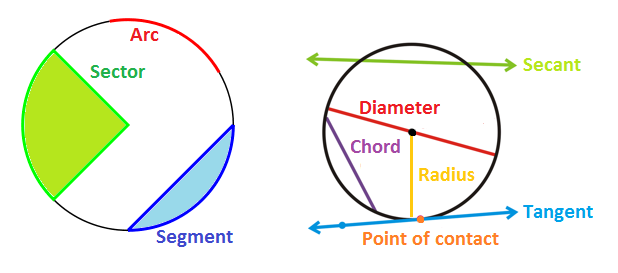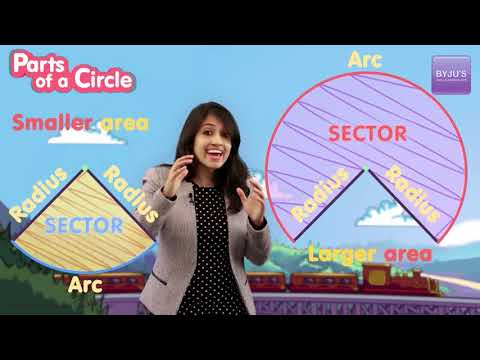Jet Set Go! All about Aeroplanes Jet Set Go! All about Aeroplanes

# Parts of a Circle

Many objects that we come across in our daily life are ‘round’ in shape such as a coin, bangles, bottle caps, the Earth, wheels etc. In layman terms, the round shape is often referred to as a circle. A closed plane figure, which is formed by the set of all those points which are equidistant from a fixed point in the same plane, is known as a circle. In other words, a circle can be described as the locus of a point moving in a plane, in such a way that its distance from a fixed point is always constant. The fixed point is called the centre of the circle and the constant distance between any point on the circle and its centre is called the radius.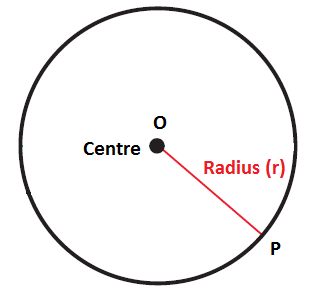Figure 1: Centre and Radius of circle figure

## Names of parts of a circle

A circle can have different parts and based on the position and shape, these can be named as follows:

• Centre
• Diameter
• Circumference
• Tangent
• Secant
• Chord
• Arc
• Segment
• Sector

As we have already discussed the centre and radius of a circle. And the diameter is equal to the twice the radius. Let us see the other parts of a circle in detail.

## Circumference of Circle

The circumference of a circle can be defined as the distance around it. This can be understood with the help of an example. Suppose a wire of length 10 cm is bent so that it forms a circle. Here, the circumference is equal to the length of the wire, i.e. 10 cm.

How to draw a circle?

Figure 1 given above, represents a circle with radius ‘r’ and centre ‘O’. A circle of any particular radius can be easily traced using a compass. The pointed leg of the compass is placed on the paper and the movable leg is revolved as shown. The traced figure gives us a circle.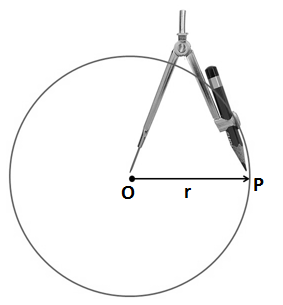Position of Points of a circle

In the below figure, various points are marked lying either outside or inside the circle or on the circle. Based on this any point can be defined as: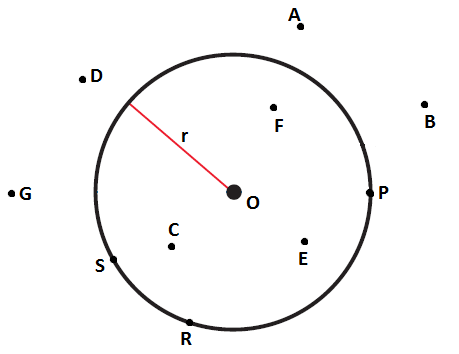1. Exterior Point: Points lying in the plane of the circle such that its distance from its centre is greater than the radius of the circle are exterior points. A point X is exterior point w.r.t to circle with centre ‘O’ if OX > r. In fig. 2 A, D, G and B are exterior points.
2. Interior Points: Point lying in the plane of the circle such that its distance from its centre is less than the radius of the circle is known as the interior point. A point X is interior-point w.r.t to circle with centre ‘O’ if OX < r. In fig. 2 C, F, and E  are interior points.
3. Points on the circumference of a circle: Points lying in the plane of the circle such that its distance from its centre is equal to the radius of a circle. In simple words, a set of points lying on the circle are points on the circumference of a circle.

A point X is said to lie on the circumference of a circle with centre ‘O’ if OX = r

In fig. 2, points P, S and R lie on the circumference of a circle and on joining these points with the centre, i.e. OR, OP and OS will represent the radius of the given circle.

Now that you have learned about a point and its relative position with respect to a circle; let’s understand a line and its relative position with respect to a circle. Given a line and a circle, it could either be touching the circle or non-touching as shown below: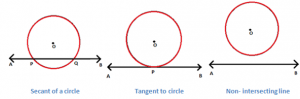Secant

In the first fig. the line AB intersects the circle at two distinct points P and Q. The line AB here is called secant of the circle. The line segment PQ is known as the chord of the circle as its endpoints lie on the circumference of the circle. A chord passing through a centre of the circle is known as the diameter of the circle and it is the largest chord of the circle.

Tangent

In the second figure, the line AB touches the circle exactly at one point, P. A line touching the circle at one single point is known as the tangent to the circle.

In the last figure, the line does not touch the circle anywhere, therefore, it is known as a non- intersecting line.

Chord

A line segment joining two different points on the circumference of a circle is called a chord of the circle. A circle can have any number of chords. Diameter is the largest chord of a circle.

## Arc of a circle

A part of a circumference of the circle is known as an arc. An arc is a continuous piece of the circle.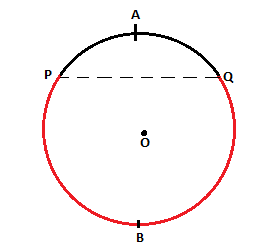The arc PAQ is known as the minor arc and arc PBQ is the major arc.

Now go through the circular region which is cut off from the rest of the circle by a secant or a chord.

Segment

Part of a circle bounded by a chord and an arc is known as a segment of the circle. The figure given below depicts the major and minor segments of the circle.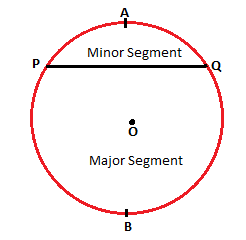Sector

A sector of a circle is the part bounded by two radii and an arc of a circle. In the below-given fig. AOB is a sector of a circle with O as centre.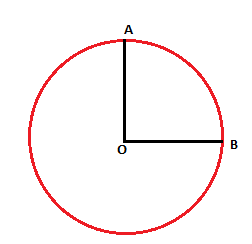### Parts of a circle diagram

The figure given below illustrates the various terms related to parts of a circle as explained above.